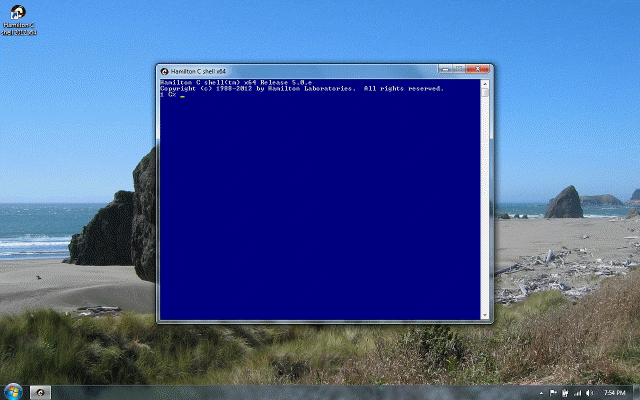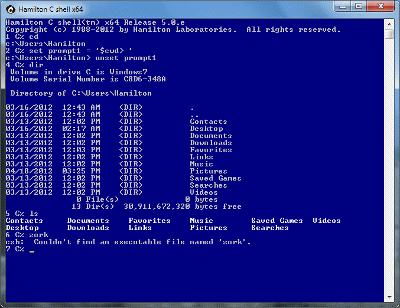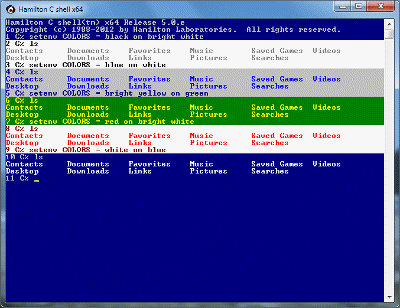## Expressions### ExpressionsPrevious | Next

#### Words versus expressions

Sometimes a character like * means wildcard and sometimes it means multiply. The meaning of what you type is determined by the context. The shell makes a distinction between words, used as arguments to a command versus an expression context.

In general, expressions are expected wherever the context would seem to suggest that it would be more natural to think of calculating a value as opposed to using wildcarding to produce a list of filenames.

#### Like C

The shell’s expression grammar is based on that of the C language and provides the full range of arithmetic, logical, bit, indexing and relation-testing and assignment operators. In addition, there are file system tests and pattern matching string compares. To use the shell as simple calculator, use the calc statement. This example shows a call to the square root routine, one of the builtin procedures.

280 D% calc sqrt(2*pi) 2.506628

which writes its result to stdout. If you want to do the calculation silently, use the @ variant:

281 D% @ r = 12 282 D% @ area = pi * r**2 283 D% calc area 452.389345

In addition to the calc and @ statements, other examples where an expression is expected include a variable index inside [...] brackets, in a procedure argument list and, as we’ll see, a number of the structured programming constructs such as the for statement.

#### Parsing

All commands are first broken down into words. A word is anything separated by a space or a tab or one of the following special strings:

& | ; > < ( ) && || >> <<

After a command line has already been broken up into words, if the context is an expression, it’s further broken up into tokens. A token is a variable or procedure name, a character or numeric literal, or one of the expression operators. Spacing between tokens is more-or-less arbitrary: for example, there’s certainly no need to put spaces around an arithmetic operator to separate it from a variable name beside it.

Tokens are separated by any of these characters or character pairs:

& | ^ + - * / % // = ! ~ < > ( ) [ ] , : ; -A -D -H -S -d -e -f -o -w -x -z ++ -- ** << >> == != =~ !~ += -= *= /= %= //= ^= **=

The <=, >=, <<=, >>=, &=, and |= operators are always broken up into separate words before expression parsing begins. For consistency, the parser will accept any of op= assignment operators with a space between the op and = parts.

Since the shell knows that any names it encounters in an expression must refer to variables or procedures it’s not necessary to use a dollar sign to introduce a variable name. In fact, you’ll find that performance is actually a bit better if you do not use a dollar sign. The reason is because a \$-style variable substitution is evaluated by converting the internal value of the variable to a string and pasting that into the expression where quite often the next step is just to convert it right back again into the integer or floating point value it started out as. Also, if floating point is involved, you may notice some loss of precision. (But don’t misunderstand, it is still perfectly legal to use \$-style variable and other substitutions in an expression.)

Character literals must appear inside single or double quotes. Numeric literals can be entered in decimal, octal or hex. Octal numbers can contain only the digits 0 through 7 and must begin with 0. Hex numbers must start with 0x and contain only 0 through f. (Either upper or lower case is acceptable.)

#### Operators

Expressions are evaluated according to the relative precedence of each operator in the expression. For example, multiplication is done before addition. The complete precedence hierarchy is shown in tabular form in the language reference.

284 D% calc 2 + 3*5 17

Some of the operators will be foreign, though we trust, not too difficult to use. The file system tests are unary operators. Each takes the name of a file or directory and tests it for existence, zero-length or some other interesting characteristic. Since the operand is a pathname, the parser temporarily shifts to word mode to read it because word mode is more natural for pathnames. The pathname can include wildcards and should not be enclosed in quotes. In the example that follows, -e tests for existence; -D tests whether the name is a directory.

285 D% cd ~\samples 286 D% ls args.c colors.csh factor.csh mcvisa.csh readme args.exe deltaday.csh finance.csh myecho.c ts.csh bits.csh dumpenv.c getprio.c myecho.exe viopaste.c bumpdate.csh dumpenv.exe getprio.exe newfiles.csh viopaste.exe caldate.csh duplicat.csh julian.csh rcode.c weekday.csh calendar.csh easter.csh makecpgm.csh rcode.exe 287 D% echo a*c args.c 288 D% calc -e a*c 1 289 D% calc -D !\$ calc -D a*c 0

#### File system tests

The value returned from a file system test is always 1 or 0; there are no restrictions on how the value might be used in further calculations.

290 D% calc 1 + (!*) + (-e myecho.c) calc 1 + ( -D a*c ) + ( -e myecho.c ) 2

#### Increment and decrement

The unary incrementing and decrementing operators are ++ and --. Pasting one in front of a variable name bumps the variable, then returns the value. Pasting one after the name bumps the variable but returns the prior state.

291 D% calc x = 1 1 292 D% calc ++x 2 293 D% calc x++ 2 294 D% calc x 3 295 D% calc --x 2

#### Bit shifting

The << and >> bit shifting operators shift an integer value on the left by the number of bit positions given by the integer value on the right. Bits shifted off the end are lost; values shifted in are always 0.

296 D% calc x << 3 8 297 D% calc x >> 10 0

#### Bitwise not

The unary ~ operator returns the bit-wise not of an integer operand. As this example shows, integers are 32-bit signed values.

298 D% calc ~5 -6 299 D% calc 0xfffffffa -6 300 D% calc ~!\$ calc ~0xfffffffa 5

#### Logical not

The unary ! operator returns the logical not. If the operand is non-zero, 0 is returned, otherwise 1. In this example, the parentheses or space after the exclamation are deliberate to avoid having the expression confused as a history reference.

301 D% calc !(5.1) 0 302 D% calc ! 0 1

#### Exponentiation

The ** operator is for exponentiation. The left operand is raised to power of the right operand.

303 D% calc 2 ** 500 3.27339061e+150

#### Modulo and integer division

The % operator is for modulo division and returns the remainder.

304 D% calc 22 % 7 1

A related // operator does integer division. Where the standard / operator might return a floating point result, // gives just the integer part of any division.

305 D% calc 8/3 2.666667 306 D% calc 8//3 2

#### Comparison operators

The == operator tests for equality; the single = means assignment. The !=, <, <=, >=, and > operators are all straight-forward tests of “not equal,” “less than,” “less than or equal,” etc. Comparisons of strings are as easy as of numbers.

307 D% calc x = 3 3 308 D% calc x == 5 0 309 D% calc "able" < "baker" 1

When the shell is asked to compare two expressions, it first tries to coerce them to numeric values. This is so that, e.g., a string containing “16” compares greater than “2” even though a simple string compare would give the opposite result.

#### Pattern matching

The =~ and !~ are the “pattern matches” and “pattern fails” tests. These are done in pretty much the same way wildcarding is done. On the right is a pattern string possibly containing wildcard characters. It’s compared against the string on the left the same way a wildcard expansion would be done except that here, comparisons are case-sensitive and where alternation appears, the match succeeds if any of the alternates matches.

310 D% calc "Now is" =~ "N*i*" 1 311 D% calc "Now is" !~ "Now is" 0 312 D% calc "Now is" =~ "n*i*" 0 313 D% calc "Now is" =~ "{n,No}*i{s,the}" 1

#### Bitwise and, xor and or

The &, ^ and | operators perform bit-wise and, xor and or operations on integer operands.

314 D% calc 5 & 4 4 315 D% calc 5 ^ 3 6 316 D% calc 5 | 3 7

#### Logical and and or

The && and || operators perform logical and and or operations:

317 D% calc 5 && 4 1 318 D% calc 0 && 4 0 319 D% calc 5 || 3 1 320 D% calc 5 || 0 1

#### The ?: Operator

The ?: trinary operator selects between two alternate expressions based on the logical true or false value of the first operand.

321 D% calc 0 ? "hello" : "goodbye" goodbye 322 D% calc (5 > 3) ? "hit" : "miss" hit

#### The {...} operator

The {...} grouping operator allows you to run a command and evaluate its result as a 1 if it succeeds or a zero if it fails. For example:

323 D% calc {echo hello} hello 1 324 D% calc {cd \nonexistent} csh: Couldn't change the current directory to '\nonexistent'. 0

#### The Op= operators

Finally, the various op= operators apply the op to the left and right operands, then assign the result to the left operand.

325 D% calc x = 2 2 326 D% calc x **= 500 3.27339061e+150 327 D% calc x 3.27339061e+150

#### Type conversions

The shell always tries to evaluate expressions “sensibly” by doing any type conversions that might seem necessary. If an integer calculation results in an overflow, the shell shifts automatically to floating point.

328 D% calc 2**30 1073741824 329 D% calc 2**200 1.606938e+060

If a character string was given but an integer is needed, the shell tries to do that conversion also. Because these conversions happen automatically, without any fanfare, the following literals all compare equal:

27 27.0 033 0x1B " 27 " ' 0x1b '

(Null strings and strings consisting only of white space are considered equal to zero. This is particularly convenient for local variables, which are initially set to null strings.)

The shell does automatic conversions to a character string format when the result is being printed. Numeric results are always shown in decimal. In this example, a procedure, the builtin square root routine, is invoked as a command; the value it returns is converted from floating point to character string and printed.

330 D% sqrt 2 1.414213

The shell also converts to a character string when you reference an array but use it as if it were not.

331 D% set x = Now is the time 332 D% cd ~\samples; myecho \$x 'myecho' 'Now' 'is' 'the' 'time' arg length = 23 characters 333 D% @ y = x 334 D% myecho \$y 'myecho' 'Now is the time' arg length = 23 characters

### Previous | NextHamilton C shell, as it first wakes up.A first few commands.You can set the screen colors to your taste.